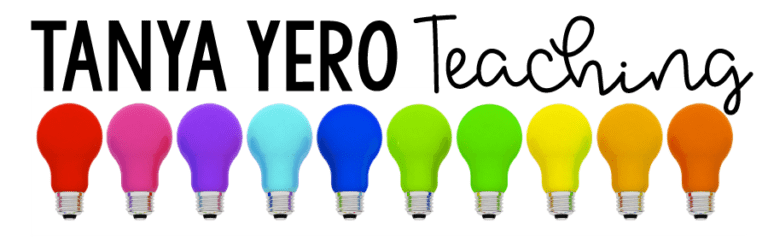# Kindergarten Word Problems Morning Work | YEARLONG Math Spiral Review Test Prep

\$13.00

Total Pages: 140
File Size: 46 MB

## Description

Visual representation is important to students. Get your students excited about math by Using Snapshots of Math Word Problems!

This purchase contains word problems for all the kindergarten grade math standards. Each word problem is applicable for young learners and includes a photo for visual representation. Each question is on a PowerPoint slide for easy use and can be used during a whole group lesson, to introduce a skill, or extra practice.

**There are 5 questions for each standard.**

Standards and Topics Covered

Operations and Algebraic Thinking
➥ K.OA.1 – Represent addition and subtraction within 10
➥ K.OA.2 – Solve addition and subtraction word problems within 10
➥ K.OA.3 – Decompose numbers less than or equal to 10
➥ K.OA.4 – Finding numbers that make 10
➥ K.OA.5 – Demonstrate fluency with addition and subtraction within 5

Number and Operations in Base Ten
➥ K.NBT.1 – Compose and decompose numbers from 11 to 19 into ten ones and some further ones.

Measurement and Data
➥ K.MD.1 – Describe measurement attributes
➥ K.MD.2 – Comparing two objects with a measurement attribute in common
➥ K.MD.3 – Classify objects into given categories

Geometry
➥ K.G.1 – Describe objects in the environment using names of shapes, and describe the relative positions of objects using positional terms.
➥ K.G.2 – Correctly name squares, circles, triangles, rectangles, hexagons, cubes, cones, cylinders, and spheres regardless of their orientations or overall size.
➥ K.G.3 – Identify squares, circles, triangles, rectangles, hexagons, cubes, cones, cylinders, and spheres as two-dimensional or three-dimensional.
➥ K.G.4 – Analyze and compare two- and three-dimensional shapes.
➥ K.G.5 – Modeling real world shapes.
➥ K.G.6 – Compose larger shapes from simple shapes.

Counting and Cardinality
➥ K.CC.1 – Know number names and recognize patterns in the counting sequence
➥ K.CC.2 – Count forward beginning from a given number within the known sequence, instead of having to begin at 1.
➥ K.CC.3 – Write numbers from 0 to 20. Represent a number of objects with a written numeral 0-20, with 0 representing a count of no objects.
➥ K.CC.4 – Understand the relationship between numbers and quantities.
➥ K.CC.5 – Count to answer “How many?” in various situations.
➥ K.CC.6 – Identify whether the number of objects, within 10, in one group is greater than, less than, or equal to the number of objects in another group, by using matching and counting strategies.
➥ K.CC.7 – Compare two numbers, within 10, presented as written numerals